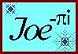Angular Unit Conversion and Circle Calculators
 Set Decimal Places: 6 1 2 3 4 5 7 8 9 10 All
 Circle Plot: Enter the radius and value of x. Click on Solve Y button to return y and angle in degrees. Circle Data Conversions: Select the known circle data from the menu, enter the value, and click on a button to convert. Angle Unit Conversions: Select the current angular unit from the menu, enter the value, and click on a button to convert. Enter a value for the radius to convert an angle to an arc length. Angle Conversion Factors: π radians = 180° = 200 grads Circular Sector and Segment Calculator: Select the sector angle or arc length from the menu and enter the value. Enter the circle radius. Arc: Portion of the circle circumference. Defined in angular units or length. Chord: The line segment connecting the endpoints of an arc. Center to Chord: Distance from the center of the circle to the midpoint of the chord. Sagitta: Balance of the distance defined above, from the midpoint of the chord to the arc. Center to Chord + Sagitta = Radius Sector Area: The area of the circle bounded by two radii and an arc. Section Area: The area of the circle bounded by the chord and the arc.Circle Plot Enter Radius = Enter x = Angle (deg.) =
 Circle Data Conversion Radius Diameter Area Circumference =